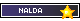(See All?) Announcements
62 Users Online

Nalda vs Ember
Print · · Subscribe · 0 Loves ·
Nalda is challenging Ember's rank, trying to dominate her HERE
Nalda is attacking first, trying to ram Ember and knock her down.

NALDA'S STATS
HP - 31
DEF - 12
ATK - 12
DMG - 5
IN - 5

EMBER'S STATS
HP - 29
DEF - 8
ATK - 5
DMG - 2
IN - 2
Code:
`[attacker="Nalda" HP="31" DEF="12" ATK="12" DMG="5" IN="5"][defender="Ember" HP="29" DEF="8" ATK="5" DMG="2" IN="2"]`

Roll Results:
Nalda rolls a d15 and gets a 10.
Nalda's' ATK: 12
12 + 10 = 22

Ember's DEF: 8
22 > 8

The attack was a HIT!

Nalda rolls a d6 and gets a 3.
Nalda's DMG: 5
5 + 3 = 8

Ember's HP: 29
29 - 8 = 21

Ember's HP: 21
Nalda's HP: 31
Because of work, my activity will fluctuate every other week. For more information and OOC preferrences check out my profileCode:
`[attacker="Ember" HP="21" DEF="8" ATK="5" DMG="2" IN="2"][defender="Nalda" HP="31" DEF="12" ATK="12" DMG="5" IN="5"]`

Roll Results:
Ember rolls a d15 and gets a 5.
Ember's' ATK: 5
5 + 5 = 10

Nalda's DEF: 12
10 < 12

The attack was a MISS!

Nalda's HP: 31
Ember's HP: 21
Because of work, my activity will fluctuate every other week. For more information and OOC preferrences check out my profileCode:
`[attacker="Nalda" HP="31" DEF="12" ATK="12" DMG="5" IN="5"][defender="Ember" HP="21" DEF="8" ATK="5" DMG="2" IN="2"]`

Roll Results:
Nalda rolls a d15 and gets a 10.
Nalda's' ATK: 12
12 + 10 = 22

Ember's DEF: 8
22 > 8

The attack was a HIT!

Nalda rolls a d6 and gets a 2.
Nalda's DMG: 5
5 + 2 = 7

Ember's HP: 21
21 - 7 = 14

Ember's HP: 14
Nalda's HP: 31
Because of work, my activity will fluctuate every other week. For more information and OOC preferrences check out my profileroll for emby? 0:)
Code:
`[attacker="Ember" HP="14" DEF="8" ATK="5" DMG="2" IN="2"][defender="Nalda" HP="31" DEF="12" ATK="12" DMG="5" IN="5"]`

Roll Results:
Ember rolls a d15 and gets a 10.
Ember's' ATK: 5
5 + 10 = 15

Nalda's DEF: 12
15 > 12

The attack was a HIT!

Ember rolls a d6 and gets a 3.
Ember's DMG: 2
2 + 3 = 5

Nalda's HP: 31
31 - 5 = 26

Nalda's HP: 26
Ember's HP: 14
Because of work, my activity will fluctuate every other week. For more information and OOC preferrences check out my profile